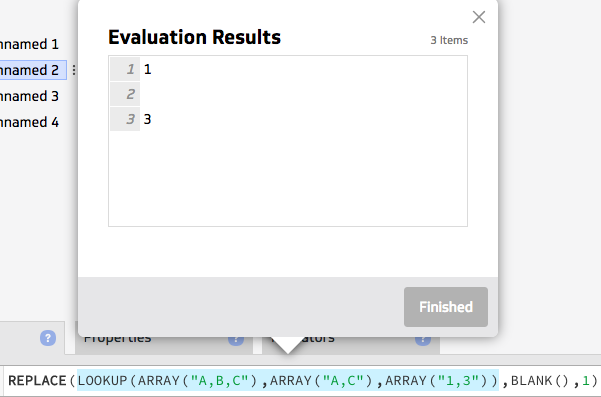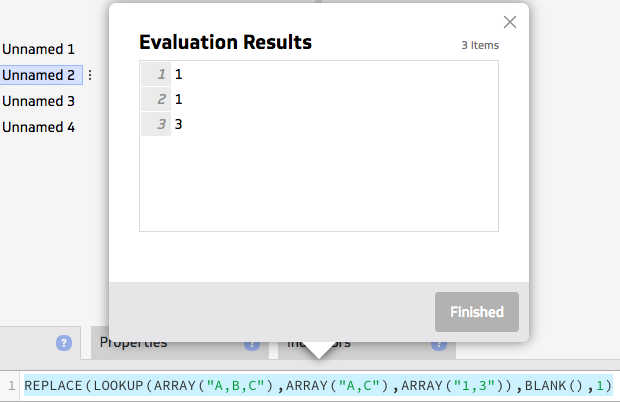In more complicated klips, I have to do some complicated lookups to return a value to perform more calculations on it.  However, I need to be able to handle instances of division by 0/blank as it will cause a chain reaction of calculations that fail.  This means often I'm writing the same complicated lookup twice.  I put it in an If Statement to determine if Blank(), and if not, then perform that complicated lookup again.  Over many calculations, this causes performance issues in Klipfolio, to the point where the klip will fail to render when a data selection is made by the user.

It occurred to me while spending time optimizing these types of calculations as much as possible, that if we had an IfBlank function, we wouldn't have to run the same complicated lookup a 2nd time when it isn't blank.  IfBlank(ComplicatedDataLookup,"0") for example.  I think this would help with function formula size and performance.

•Magne Lauritzen

This would be useful.

A quick fix in your case might be to use MAP to first perform the lookup, assign it to a variable, and then do an IF on the variable.

I made an example. You can rename the variable "val" anything you want of course.

MAP(<lookup here>, "val", if(\$val=BLANK(), "val is blank", \$val))

This function will return "val is blank" if the return of the lookup is blank, or return the result of the lookup otherwise.

•Randy Fullmer

If variables supported arrays...  But that's another feature request.

•Seth Epps

Hey Randy,

Could you give an example of what's being returned by your LOOKUP to understand how best to handle the particular cases? If you're running into issues with the divide by 0/blank returning "Infinity", could using REPLACE(<data>, "Infinity","some value") be a possible alternative?

-Seth

•Randy Fullmer

Replace was not something I had considered for dealing with Infinity and NaN, but it appears to work for some situations, however, I still think an IFBlank would be helpful.

This is a very basic example, and the actual formula is far more complex, and the klip uses many similar calculations, which are then referenced by other components for additional calculations, which can be a performance issue.

`Lookup(!SalesReps,A:A,B:B)returns something like:.45.1.4{blank}.7.15`
`Simplified Formula:(1-Lookup(!SalesReps,A:A,B:B))/max(1-Lookup(!SalesReps,A:A,B:B))Results:.55.9.61.3.85`

Issue:
The lookup finding blank() treated it as 0, which caused all results to be incorrect. The blank shouldn't even be factored into the equation for my requirement.
I need to create logic to not utilize the blank. These are the results I'd want in the end:
.611
1
.666
0
.333
.944

Replace doesn't appear to work with Blank(), which makes sense.
I need to return 0 if the lookup value is blank.

workaround using Replace (better than I was doing before):

`Replace( (1-Lookup(!SalesReps,A:A,B:B)) / Max(    1-    IF(       Lookup(!SalesReps,A:A,B:B)=blank(),       1,       Lookup(!SalesReps,A:A,B:B)    ) ), "Infinity", 0)`

However...
Suggestion:

`Replace( (1-Lookup(!SalesReps,A:A,B:B)) / Max(1-IFBLANK(Lookup(!SalesReps,A:A,B:B)),1)), "Infinity", 0)`

That's one less lookup that needs to be performed, and over a large klip doing similar things like this many times, where those lookups are actually using complicated select statements... every little bit helps when it comes to performance.

However, I'm sure there must be even better ways. I've modified datasources to try and help with these scenarios the best I can. The IfBlank() function idea came to me when I was working with ISBLANK() in Excel, and wondered why there wasn't anything similar here. Thank you for the suggestion of using Replace.

•Seth Epps

"Replace doesn't appear to work with Blank(), which makes sense."

This isn't necessarily true; if your IF function,

`IF(       Lookup(!SalesReps,A:A,B:B)=blank(),       1,       Lookup(!SalesReps,A:A,B:B)    )`

is working, the REPLACE should work as well (See the below images for an example).This should reduce your formula to

`Replace( (1-Lookup(!SalesReps,A:A,B:B)) / Max(    1- Replace(Lookup(!SalesReps,A:A,B:B),blank(),1) ), "Infinity", 0)`

I can see the above formula failing if the blank space is not a result of the LOOKUP, but rather an empty string in the B:B data. In that case, you could cover all your bases by passing an array of values you'd like to replace with "1". For example,

`Replace(Lookup(!SalesReps,A:A,B:B),array(blank(),""),1)`

-Seth

•Randy Fullmer

Interesting.  I had tested Replace with blank before and it was not working, but I just checked again, in and outside of an array, and it seems to work.  So ok then.

I control the data, so I won't return empty strings.

Thanks for your assistance on providing a workaround, and I'll see if I can apply this type of logic to the klips I'm working on (but I still think an IfBlank/IsBlank would be an intuitive useful function)